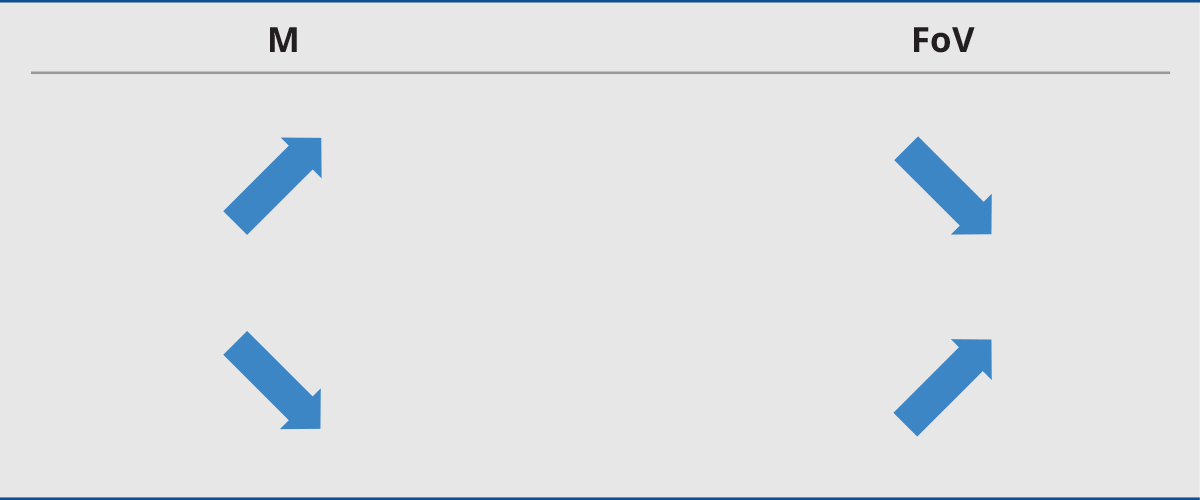## Magnification and field of view

The magnification M of an optics describes the ratio between image (h’) and object size (h)

M = h^’ / h

A useful relationship between working distance (s), magnification (M) and focal length (f) is the following:

s = f(M-1)/MMacro and telecentric lenses are designed to work at a distance comparable to their focal length (finite conjugates), while fixed focal length lenses are designed to image objects located at a much greater distance than their focal length (infinite conjugates). It is thus convenient to classify the first group by their magnification, which makes it easier to choose the proper lens given the sensor and object size, and the latter by their focal length.

Since fixed focal length lenses also follow the previous equation, it is possible to calculate the required focal length given the magnification and working distance, or the required working distance given the sensor size, field of view and focal length, etc. (some examples are given at the end of this section). For macro and telecentric lenses instead, the working distance and magnification are typically fixed.

Next →